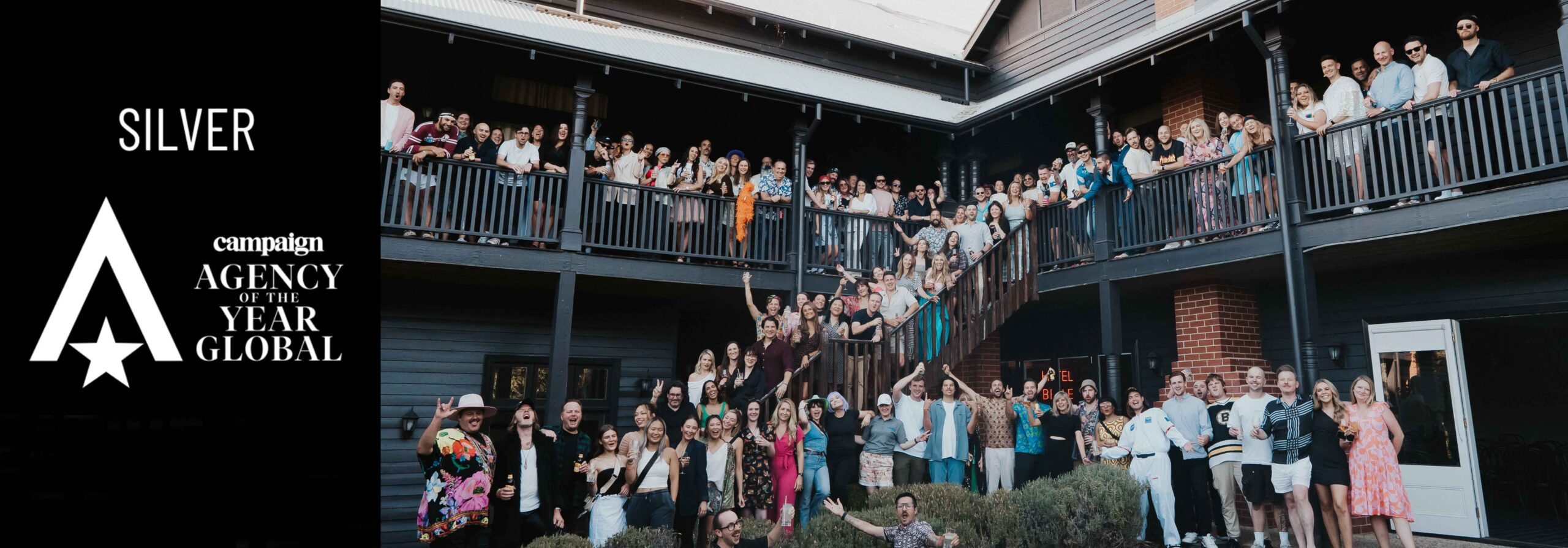# Thinkerbell ranked number 2 Global Creative Agency of the Year by UK’s CampaignBringing home some silverware.

Wow. At the UK’s Campaign Global Agency of the Year Awards, we’ve ranked at number 2! We’re stoked to have brought home Silver and seeing the work we are doing down under recognised on the global stage. Our Measured Magic is spreading…

Let’s talk. give us a bell.
Let’s talk. give us a bell.
Let’s talk. give us a bell.
Let’s talk. give us a bell.
Let’s talk. give us a bell.
Let’s talk. give us a bell.
Let’s talk. give us a bell.
Let’s talk. give us a bell.
Let’s talk. give us a bell.
Let’s talk. give us a bell.
Let’s talk. give us a bell.
Let’s talk. give us a bell.
Let’s talk. give us a bell.
Let’s talk. give us a bell.
Let’s talk. give us a bell.
Let’s talk. give us a bell.
Let’s talk. give us a bell.
Let’s talk. give us a bell.
Let’s talk. give us a bell.
Let’s talk. give us a bell.